Courses

# RS Aggarwal Solutions: Whole Numbers Exercise - 3E Notes | EduRev

## Class 6 : RS Aggarwal Solutions: Whole Numbers Exercise - 3E Notes | EduRev

The document RS Aggarwal Solutions: Whole Numbers Exercise - 3E Notes | EduRev is a part of the Class 6 Course Mathematics (Maths) Class 6.
All you need of Class 6 at this link: Class 6

Q.1. Divide and check your answer by the corresponding multiplication in each of the following :
(i) 1936/16
(ii) 19881/47
(iii) 257796/341
(iv) 612846/582
(v) 34419/149
(vi) 39039/1001
Ans. (i) By actual division, we have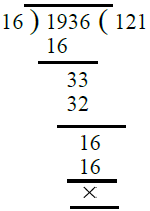Check : 121 × 16 = 1936.
(ii) By actual division, we have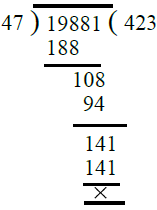Check :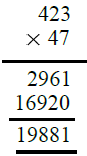(iii) By actual division, we have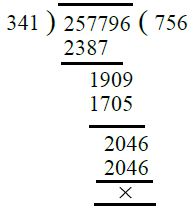Check :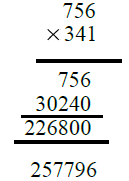(iv) By actual division, we have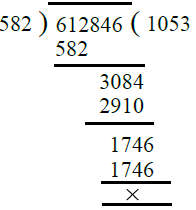Check :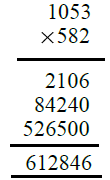(v) By actual division, we have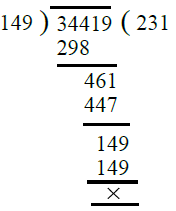Check :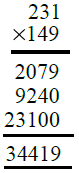(vi) By actual division, we have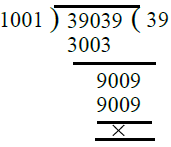Check :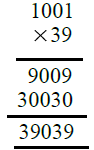Q.2. Divide and find out the quotient and remainder. Check your answer :

(i) 6971/47
(ii) 4178/35
(iii) 36195/153
(iv) 93575/400
(v) 23025/1000
(vi) 16135/875
Ans. (i) By actual division, we have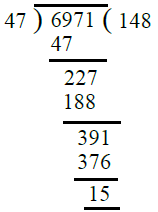∴ Quotient = 148, Remainder = 15
Check : Dividend = Divisor × Quotient + Remainder
= 47 × 148 + 15
= 6956 + 15
= 6971
(ii) By actual division, we have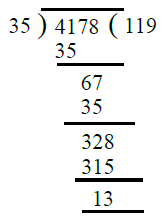∴ Quotient = 119, Remainder = 13
Check : Dividend = Divisor × Quotient + Remainder
= 35 × 119 + 13
= 4165 + 13
= 4178
(iii) By actual division, we have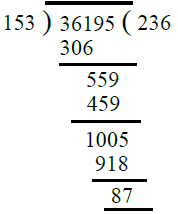∴ Quotient = 236, Remainder = 87
Check : Dividend = Divisor × Quotient + Remainder
= 153 × 236 + 87

= 36108 × 87
= 36195
(iv) By actual division, we have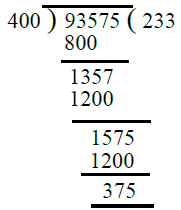∴ Quotient = 233, Remainder = 375
Check : Dividend = Divisor × Quotient + Remainder

= 400 × 233 + 375
= 93200 × 375
= 93575
(v) By actual division, we have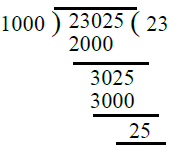∴ Quotient = 23, Remainder = 25
Check : Dividend = Divisor × Quotient + Remainder
= 1000 × 23 + 25
= 23000 × 25
= 23025
(vi) By actual division, we have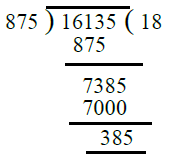∴ Quotient = 18, Remainder = 385
Check : Dividend = Divisor × Quotient + Remainder
= 875 × 18 + 385

= 15750 × 385
= 16135

Q.3. Find the value of :
(i) 65007/1
(ii) 0/879
(iii) 981 + 5720/10
(iv) 1507 – 625/25
(v) 32277/(648 – 39)
(vi) 1573/1573 – 1573/1573
Ans.
(i) We know that any number (non-zero)
divided by 1 gives the number itself
∴ 65007/1 = 65007
(ii) We know that 0 divided by any natural
number gives 0
∴ 0/879 = 0
(iii) 981 + 5720/10 = 981 + (5720/10)
= 981 + 572
= 1553
(iv) 1507 – 625 25 = 1507 – (625 25)
= 1507 – 25
= 1482
(v) 32277/(648 – 39)
= 32277/609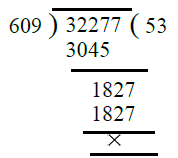∴ 32277/(648 – 39) = 53
(vi) 1573/1573 – 1573/1573
= (1573/1573) – (1573/1573)
= 1 – 1 = 0

Q.4. Find a whole number n such that n/n = n.

Ans. We have n/n = n
let n = 1, 1/1 = 1

1 = 1
which is true
Hence 1 is the required whole number.

Q.5. The product of two numbers is 504347. If one of the numbers is 317, find the

other.
Ans. Product of two numbers = 504347
One number = 317
∴ Other number = 504347/317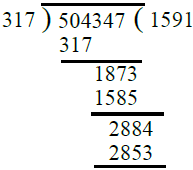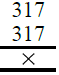∴ Other number = 1591

Q.6. On dividing 59761 by a certain number, the quotient is 189 and the remainder is 37. Find the divisor.
Ans.
Here Dividend = 59761, Quotient = 189
Remainder = 37
We know that Dividend = Divisor × Quotient + Remainder
∴ 59761 = Divisor × 189 + 37
59761 – 37 = Divisor × 189
59724 = Divisor × 189
Divisor × 189 = 59724
Divisor = 59724/189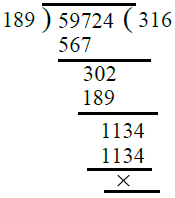∴ Divisor = 59724/189 = 316

Q.7. On dividing 55390 by 299, the remainder is 75. Find the quotient, using the division algorithm.

Ans.
Here dividend = 55390,

Divisor = 299 and Remainder = 75
By division algorithm, we have
Dividend = Quotient × Divisor + Remainder
∴ 55390 = Quotient × 299 + 75
55390 – 75 = Quotient × 299
55315 = Quotient × 299
Quotient × 299 = 55315
∴ Quotient = 55315/299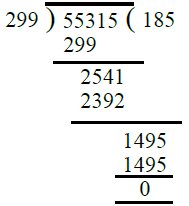∴ Required quotient = 185

Q.8. What least number must be subtracted from 13601 to get a number exactly divisible by 87 ?
Ans.
On dividing 13601 by 87, we have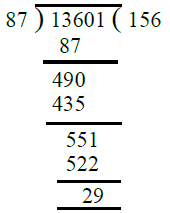It is clear that if we subtract 29 from 13601, the resulting number will be exactly divisible by 87.
∴ The required least number = 29.

Q.9. What least number must be added to 1056 to get a number exactly divisible by 23 ?
Ans.
Here dividend = 1056, Divisor = 23
By actual divison, we have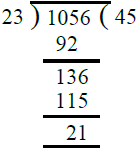It is clear that if we add 2 to 21, it will become 23 which is divisible by 23.
∴ Required least number = 2.

Q.10. Find the largest 4-digit number divisible by 16.
Ans.
Greatest 4-digit number = 9999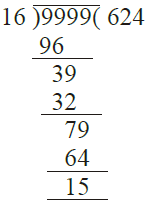On, dividing by 16, we get remainder as 15
∴ The required largest 4-digit number
= 9999 – 15 = 9984

Q.11. Divide the largest 5-digit number by 653. Check your answer by the division algorithm.
Ans. Largest number of 5-digits = 99999
On dividing 99999 by 653, we have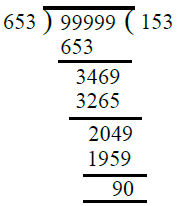∴ Quotient = 153, Remainder = 90

Check : By division algorithm
Dividend = Divisor × Quotient + Remainder
= 653 × 153 + 90
= 99909 + 90
= 99999

Q.12. Find the least 6-digit number exactly divisible by 83.
Ans. The least 6-digit number = 100000
On dividing 100000 by 83, we have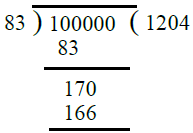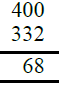It is clear that if we add 15 to 68, it will become 83 which is divisible by 83.
∴ Required least 6-digit number
= 100000 + 15 = 100015

Q.13. 1 dozen bananas cost Rs. 29. How many dozens can be purchased for Rs. 1392 ?
Ans. Cost of 1 dozen bananas = Rs. 29
∴ Bananas can be purchase in Rs. 1392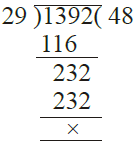= 1392/29 = 48 dozens

Q.14. 19625 trees have been equally planted in 157 rows. Find the number of trees in each row.
Ans. Total number of trees = 19625

Total number of rows = 157
∴ Number of trees in each row
= 19625/157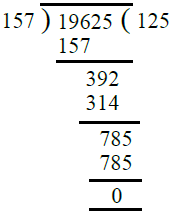∴ Number of trees in each row = 125

Q.15. The population of a town is 517530. If one out of every 15 is reported to be

literate, find how many literate persons are there in the town.
Ans. Total population of the town = 517530 Since there is one educated person out

of 15.
∴ Total number of educated persons in the town = 517530/15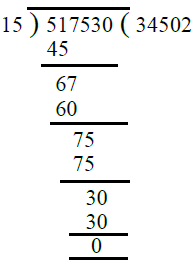∴ Total number of educated persons in the town = 34502.

Q.16. The cost price of 23 colour television sets is Rs. 570055. Determine the cost price of each TV set if each costs the same.
Ans.
Cost of 23 colour TV sets = Rs. 570055
Cost of 1 colour TV set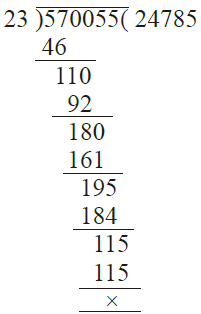∴ Cost of 1 colour TV set
= Rs. 570055/23 = Rs. 24785

Offer running on EduRev: Apply code STAYHOME200 to get INR 200 off on our premium plan EduRev Infinity!

## Mathematics (Maths) Class 6

191 videos|224 docs|43 tests

,

,

,

,

,

,

,

,

,

,

,

,

,

,

,

,

,

,

,

,

,

;